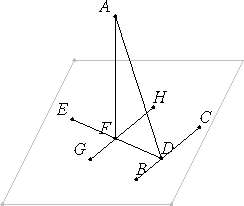# Proposition 11

To draw a straight line perpendicular to a given plane from a given elevated point.

Let A be the given elevated point, and the plane of reference the given plane.

It is required to draw from the point A a straight line perpendicular to the plane of reference.I.12

Draw any straight line BC at random in the plane of reference, and draw AD from the point A perpendicular to BC.

Then if AD is also perpendicular to the plane of reference, then that which was proposed is done.

But if not, draw DE from the point D at right angles to BC and in the plane of reference, draw AF from A perpendicular to DE, and draw GH through the point F parallel to BC.

XI.4

Now, since BC is at right angles to each of the straight lines DA and DE, therefore BC is also at right angles to the plane through ED and DA.

XI.8

And GH is parallel to it, but if two straight lines are parallel, and one of them is at right angles to any plane, then the remaining one is also at right angles to the same plane, therefore GH is also at right angles to the plane through ED and DA.

XI.8

And GH is parallel to it, but if two straight lines are parallel, and one of them is at right angles to any plane, then the remaining one is also at right angles to the same plane, therefore GH is also at right angles to the plane through ED and DA.

XI.Def.3

Therefore GH is also at right angles to all the straight lines which meet it and are in the plane through ED and DA.

But AF meets it and lies in the plane through ED and DA, therefore GH is at right angles to FA, so that FA is also at right angles to GH. But AF is also at right angles to DE, therefore AF is at right angles to each of the straight lines GH and DE.

But if a straight is set up at right angles to two straight lines which cut one another at their intersection point, then it also is at right angles to the plane through them. Therefore FA is at right angles to the plane through ED and GH.

XI.4

But the plane through ED and GH is the plane of reference, therefore AF is at right angles to the plane of reference.

Therefore from the given elevated point A the straight line AF has been drawn perpendicular to the plane of reference.

Q.E.F.

## Guide

In the proof, before the line AD can be drawn from the point A perpendicular to the line BC, it is necessary to know that the point and line belong to the same plane. Such a plane can be specified by taking the line BC and a line from A to any point on BC since two intersecting lines determine a plane (XI.2).

#### Use of this proposition

The construction in this proposition is used frequently in the last three books of the Elements.